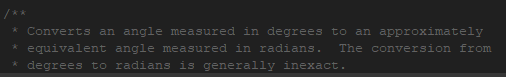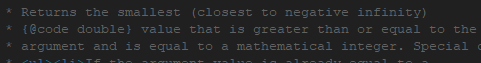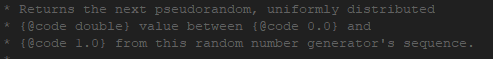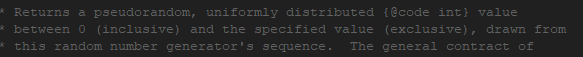# Math类中有根据传入的参数类型不同

Math中还包含一些静态常量供我们调用。

1.abs()，返回该数的绝对值。

``````public class Test {
public static void main(String[] args) {
System.out.println(Math.abs(1.0));
System.out.println(Math.abs(-1));
System.out.println(Math.abs(-0));
}
}

1.0
1
0
``````

Math类中有根据传入的参数类型不同，设置了多个abs函数用于接收不同的类型的参数，并返回对应类型的绝对值。2.三角函数（sin,cos,tan）``````public class Test {
public static void main(String[] args) {
System.out.println(Math.sin(30 * (Math.PI / 180.0)));//1度 = PI / 180弧度，
}                                                     //传进去的参数作为角度，然后乘以PI / 180

}
``````

``````运行结果：
0.49999999999999994
0.49999999999999994
``````

sin30度应该是0.5，计算机算的有一定的误差。

3.反三角函数（asin()，acos() , atan()）。

``````public class Test {
public static void main(String[] args) {
System.out.println(Math.asin(0.5) / (Math.PI / 180.0));//将返回的弧度，转化为角度。
System.out.println(Math.toDegrees(Math.asin(0.5)));   //通过toDgrees转换
}
}

30.000000000000004
30.000000000000004
``````

4.sqrt() 开平方

``````public class Test {
public static void main(String[] args) {
System.out.println(Math.sqrt(2));  // √2
System.out.println(Math.sqrt(3));  // √3
System.out.println(Math.sqrt(4));
}
}

1.4142135623730951
1.7320508075688772
2.0
``````

5.pow(double a, doubel b),求a^b

``````public class Test {
public static void main(String[] args) {
System.out.println(Math.pow(1, 100)); // 1^100
System.out.println(Math.pow(2, 3));
System.out.println(Math.pow(5, 2.5)); //5^2.5
}
}

1.0
8.0
55.90169943749474
``````

6.ceil（）``````public class Test {
public static void main(String[] args) {
System.out.println(Math.ceil(-1.2));//往数值较大的整数靠齐，-1 比 -1.2大
System.out.println(Math.ceil(-0.5));//0比-0.5大。
System.out.println(Math.ceil(1));
System.out.println(Math.ceil(1.2));
System.out.println(Math.ceil(1.5));
}
}

-1.0
-0.0
1.0
2.0
2.0
``````

7.floor()``````public class Test {
public static void main(String[] args) {
System.out.println(Math.floor(-1.2));//往数值较小的整数靠齐，-2比 -1.2小
System.out.println(Math.floor(-0.5));//0比-0.5大。
System.out.println(Math.floor(1));
System.out.println(Math.floor(1.2));
System.out.println(Math.floor(1.5));
}
}

-2.0
-1.0
1.0
1.0
1.0
``````

8.round()

``````public class Test {
public static void main(String[] args) {
System.out.println(Math.round(-2.8)); //-3
System.out.println(Math.round(-2.6)); //-3
System.out.println(Math.round(-2.5)); //-2
System.out.println(Math.round(-1.5)); //-1
System.out.println(Math.round(-1.4)); //-1
System.out.println(Math.round(-0.4)); // 0
System.out.println(Math.round(0.4));  // 0
System.out.println(Math.round(1.4));  //1
System.out.println(Math.round(1.5));  //2
System.out.println(Math.round(2.5));  //3
System.out.println(Math.round(2.6));  //3
System.out.println(Math.round(2.8));  //3
}
}

-3
-3
-2
-1
-1
0
0
1
2
3
3
3
``````

还有一些方法就不一 一解释了，有兴趣的可以查阅文档或者看源码及其注释。

1. nextInt()和nextInt(int bound)

nextInt（）是直接生产int范围内的伪随机数，有2^32种可能。

nextInt(int bound) 生成 [0,boudn) 范围之间的伪随机数，包含0，不包含bound。

``````public class Test {
public static void main(String[] args) {
Random r = new Random();
for(int i = 0; i < 3; i  ){
System.out.println(r.nextInt());//生成随机数
}
for(int i = 0; i < 3; i  ){
System.out.println(r.nextInt(10));//生成[0,10)之间的随机数，包含0，不包含10.
}
}
}

442673013
19803501
-388344272
0
2
7
``````

2.nextfloat（）与nextdouble（）类似，只是返回值类型不同。

nextFloat()返回0.0f~1.0f之间的随机数。

nextDouble（）返回0.0 ~ 1.0之间的随机数。（小数默认是double精度）

nextfloat（）与nextdouble（）源码里面两个方法的注释只写了取0.0~1.0之间的随机数，没有说两端的情况。netxDouble()和nextFlaot()注释，0.0~1.0之间（between）